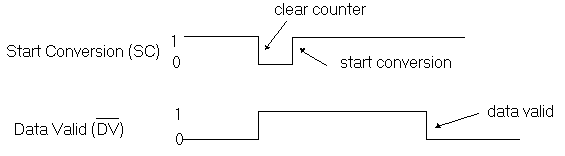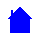The Tracking, Ramp or Staircase type of Analog to Digital Converter uses a counter and a DAC (digital to Analog converter) to match the digital output to the analog input. It does this by converting the sequential count back into an analog signal and comparing the voltage level to the input signal., stopping the count when the two are equal. With this method of conversion, the output climbs from zero to the desired value and it is going to take longer to produce a correct output for higher voltages than for lower voltages. Because it is an ASYNCHRONOUS event handshaking signals are necessary between the controlling device and the ADC and these are given below. Also, as it takes a varying amount of time to convert the analog signal, a Sample and Hold circuit is required at the input so that the voltage level can be held steady long enough for it to be converted, a sample and hold circuit is a circuit that "grabs" a varying input at some point in time and presents the analog level at that instant at it's output, it holds this level until commanded to "sample" the input signal again. Finally, the resolution is the change in voltage that occurs when the least significant bit is changed (e.g counting from a "1" to a "2" or from "0" to "1")

Diagram of a Tracking ADCTiming Diagram for control signals to the ADCResolution for an ADCSample and Hold diagramELET1215 Homepage